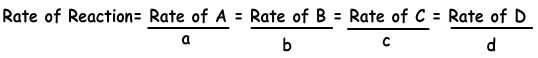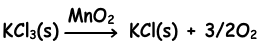## Rates of Reactions (Chemical Kinetics) Cheat Sheet

Rates of Reactions (Chemical Kinetics) Cheat Sheet

Rates of Reactions (Chemical Kinetics)

Physical, chemical and nuclear reactions take place in different speeds. Chemical rate is the amount of change in the matter in unit time.

Reaction Rate=(Change in amount of matter)/time

Δ[A(g)] is the representation of change in molarity of A gas. Reaction rate can be written for all matters in the reaction.

Following equation shows reaction rate in terms of products and reactants.

aA + bB → cC + dDActivation Energy

It is the energy required to start chemical reaction. Reactions having higher activation energy has lower reaction rate. Activation energy can only be changed by catalysts. They decreases activation energy of reaction and increase rate of reaction.MnO2 is the catalyst of this reaction.

Factors Affecting Reaction Rate

1. Types of matter in reaction:

• In general reactions having reactants and products in gas phases have higher reaction rate than reactions consisting of matters in liquid phase.
• Breaking too many bonds and forming new ones makes reaction slow down.
• Reactions consisting of ions having opposite signs have high reaction rate.

2. Concentration of matters in reaction:

Collision theory is the most successful one that explains chemical reaction. Particles must collide to react each other. On the contrary, all collisions do not result with reaction.

• Matters should have required energy to react.
• Particles should collide in appropriate geometry to react.

Relation between concentrations of matters and reaction rate can be explained like;

aA  + bB cC + dD

Reaction rate of this reaction is found by;

Reaction Rate = k. [A]a.[B]b

Where; k is the rate constant or rate coefficient that depends on temperature and a and b are exponents.

Order of Reaction;

Order of reaction is the sum of exponents. For example;

Reaction Rate = k. [A]2.[B].[C]3 order of this reaction is 2+1+3=6.

3.Temperature in Reactions:

Increasing in the temperature about 10 0C, results increase in the reaction rate. When we increase temperature;

• number of collisions in unit time increases
• number of particles having energy above activation energy increases.

4. Catalysts:
Catalysts are matters that increase or decrease reaction rate and they do not being changed in reaction.

5. Surface of Reactants:

If size of particles are small, then surface of them increases. Increasing surface of the reactants, increase reaction rate.

Rates of Reaction Exams and Problem Solutions

Related